## Calculate Days Open in Excel

If you want to know how to Calculate days in Excel, there are some formulas that you can use to do so. For example, you can use the DAYS function in Excel to find the number of days between two dates. However, if you want to find the number of days a ticket has been opened, you can use the if function.

This function calculates the number of days between two dates. It requires the date to be in an Excel-compatible format. Using the IF function, you can also specify the year, month, and day.

## 1. Calculate the Number of Days a Ticket Has Been Opened in Excel

It is important to track the time it takes to resolve support tickets whenever you are dealing with them. You can use Excel’s built-in function to calculate the backlog to do this. When your team members work long hours, the number of tickets they must resolve each day can increase significantly. In order to calculate the backlog, you first need to know the average resolution time of tickets. Once you have the average resolution time, you can subtract the open date from the current date to calculate the days a ticket has been open.

To calculate how many days a ticket has been open, you must first determine the date when the ticket was authored. You can also find out the number of days between a ticket’s date of issuance and its closing date by adding up the days between the two dates. Here’s an example. The ticket was created on April 18, 2022, and is still open. You can also calculate the number of days the ticket has been open using Excel. The formula is easy to use and makes analyzing your data easier.

``=IF(ISBLANK(D5),TODAY()-C5,D5-C5)``

If you want to calculate the number of days the spreadsheet has been open, you can use the IF function. In this case, you will need to input a date in a format that Excel understands.

## 2. Conclusion

You can use the IF function to Calculate the number of days a ticket remains open. You can enter the values in the formula, and a calculation will display the number of days that have passed since it was opened. The result of this calculation will be the number of days between the original ticket creation date and the date it was last reopened. By just putting in the values in the manner mentioned above, you can calculate the days opened!

## 3. Related Functions

• Excel ISBLANK function
The Excel ISBLANK function returns TRUE if the value is blank or null.The syntax of the ISBLANK function is as below:= ISBLANK (value)…
• Excel IF function
The Excel IF function perform a logical test to return one value if the condition is TRUE and return another value if the condition is FALSE. The IF function is a build-in function in Microsoft Excel and it is categorized as a Logical Function.The syntax of the IF function is as below:= IF (condition, [true_value], [false_value])….
• Excel TODAY function
The Excel TODAY function returns the serial number of the current date. So you can get the current system date from the TODAY function. The syntax of the TODAY function is as below:=TODAY()…
• Excel DAYS function
The Excel DAYS function returns the number of days between two dates.The syntax of the DAYS function is as below:= DAYS (end_date,start_date)…

## Add Row Numbers And Skip Blanks in Excel

Do you ever have to input a list of numbers into a spreadsheet, and some of the cells are blank? It can be difficult to keep track of what number is in which cell when you have to scroll up and down the sheet to look for the right spot. Well, today, I’m going to show you how to add row numbers and skip blanks so that your data entry process is a little bit smoother. Let’s get started!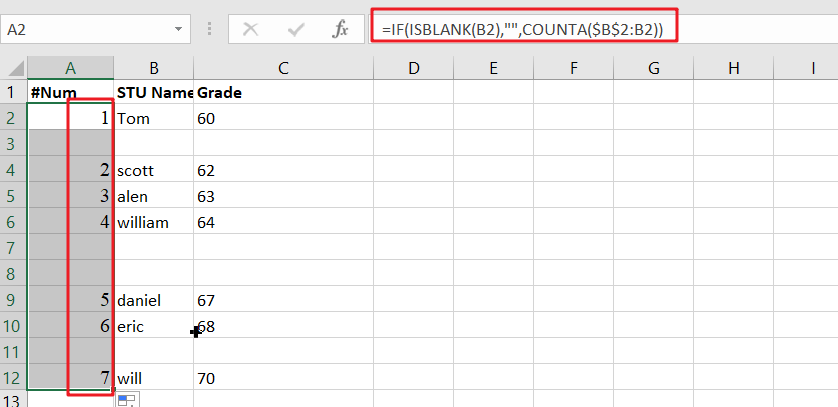## Generic formula

`=IF(ISBLANK(B2),"",COUNTA(\$B \$2: B 2))`

## Summary

To add row numbers to a list of data, skipping blank cells, you can use the formula based on COUNTA and ISBLANK. Just using the following formula:

`=IF(ISBLANK(B2),"",COUNTA(\$B\$2:B2))`A simple way is to use IF statements with anondata formulas to show certain information when it comes up while keeping other things hidden from view unless desired by users who will open them up specifically looking for these details themselves!

## Explanation

The purpose is to add row numbers in column A only when there’s a value present elsewhere.

The IF function checks whether or not cell B2 has any values with ISBLANK.

`=ISBLANK(B2) // FALSE if not, TRUE if empty`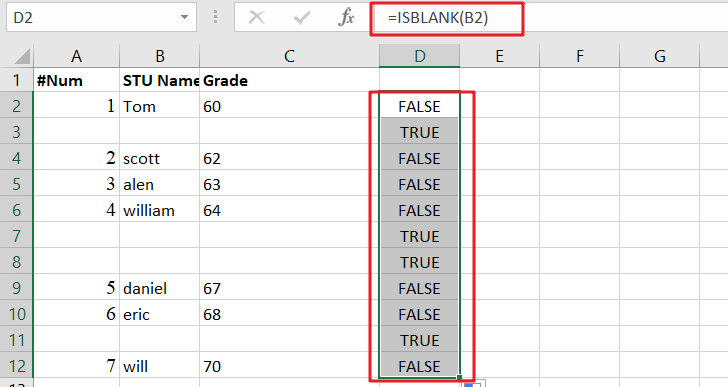When B2 is empty, ISBLANK returns TRUE, and the IF function produces a plain string like this: “”. If there’s anything in between them (i.e., not an expandable reference), we get COUNTA with whatever values are stored at each cell of that array; namely, nothing if it exists or NaN for non-numbers.

`=COUNTA(\$B\$2:B2) // expanding range`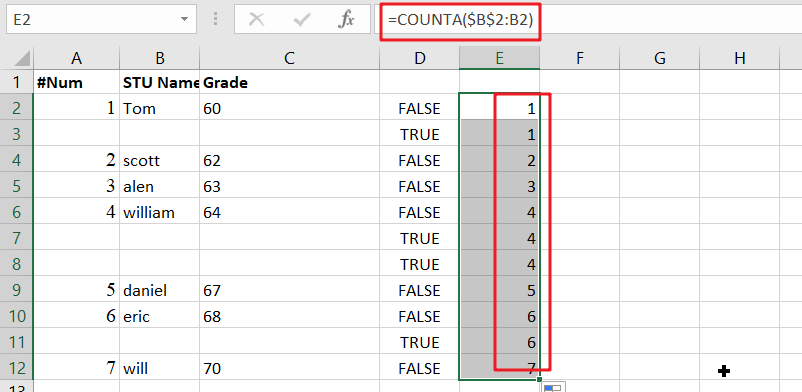As the formula is copied down, each row’s count expands to include all non-blank cells in that range. COUNTA will count both numbers and text, making it easy for you to determine who was counting what during your data entry!

## Method2: Autonumber Rows If Adjacent Cell Not Blank

The easiest way to auto number your column is by using this formula: =IF(B2<>””,COUNTA(\$B\$2:B2),””) and then dragging down with the filehandle. You can also add row numbers manually if you want, but why not make things easier on yourself?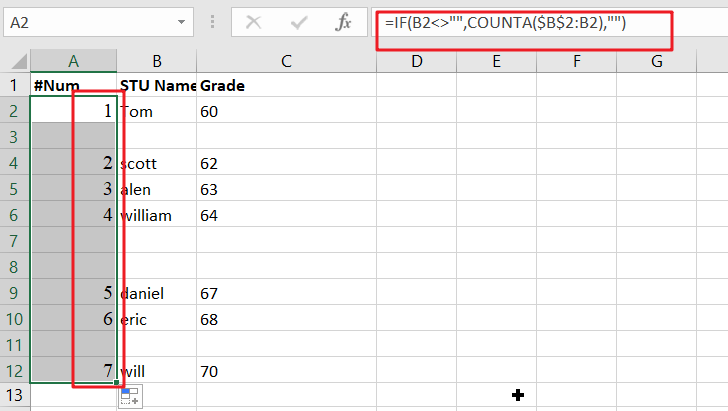Note: When you enter a formula to auto number cells, make sure that B2 is populated with the relevant value.

Entering new data or deleting existing values will automatically cause the sequence number in column A to be renumbered.

### Related Functions

• Excel ISBLANK function
The Excel ISBLANK function returns TRUE if the value is blank or null.The syntax of the ISBLANK function is as below:= ISBLANK (value)…
• Excel IF function
The Excel IF function perform a logical test to return one value if the condition is TRUE and return another value if the condition is FALSE. The IF function is a build-in function in Microsoft Excel and it is categorized as a Logical Function.The syntax of the IF function is as below:= IF (condition, [true_value], [false_value])….
• Excel COUNTA function
The Excel COUNTA function counts the number of cells that are not empty in a range. The syntax of the COUNTA function is as below:= COUNTA(value1, [value2],…)…

## Check If a Cell is Blank or Empty

The article demonstrates how to check if a range of cells is blank or empty by ISBLANK and other functions to recognize empty cells in Excel and take appropriate action based on their status.

There are several circumstances in which you need to determine if a cell is empty or not. If a cell is blank, for example, you may want to add, count, copy a value from another cell, or do nothing. ISBLANK is the appropriate function to employ in these situations, occasionally alone, but most often in conjunction with other Excel functions.

## ISBLANK in Excel – a few points to keep in mind

The critical item to remember is that the ISBLANK function in Excel recognizes actually empty cells, that is, cells that contain nothing: no spaces, no tabs, no carriage returns, or anything else that looks blank in a view.

ISBLANK returns FALSE for a cell that seems to be blank but is not. If a cell includes any of the following, this behavior occurs:

`=IF(A1>"", A1, "") //yields an empty string`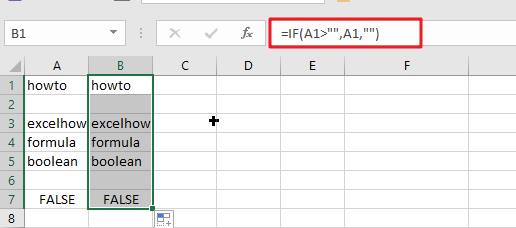Imported zero-length string from an external database or as a consequence of a copy/paste operation.

Spaces, apostrophes, non-breaking spaces (&nbsp);, linefeeds, and other non-printing characters are permitted.

## How to utilize the ISBLANK function in Excel

To obtain a better grasp of the ISBLANK function‘s capabilities, consider these practical applications.

### If a cell is blank in Excel, then

Because Microsoft Excel does not have an IFBLANK function, you must use IF and ISBLANK to test a cell and execute an action if it is empty.

The general version is as follows:

`=IF(ISBLANK(B1), "open", "completed")`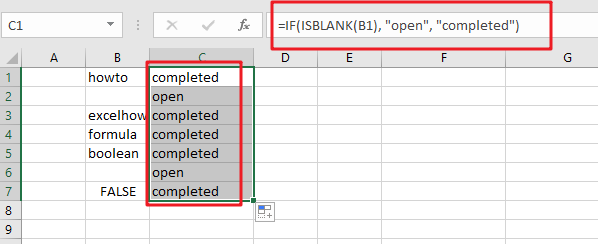To demonstrate, let’s verify whether a cell in column B (delivery date) has any data. If the cell is empty, output “Open”; if it is not empty, produce “Completed”.

Please keep in mind that the ISBLANK function only returns cells that are completely blank. ISBLANK returns FALSE if a cell contains anything invisible to the human eye, such as a zero-length string.

### If any cell in range is blank, then do something

There are many techniques to check a range of cells in Microsoft Excel for empty cells. We will use an IF statement to output one value if the range has at least one empty cell and another value if the range contains no empty cells. The logical test is performed by calculating the total number of empty cells in the range and then determining if the count is larger than zero. This may be accomplished via the use of the COUNTBLANK or COUNTIF functions.

### Related Functions

• Excel ISBLANK function
The Excel ISBLANK function returns TRUE if the value is blank or null.The syntax of the ISBLANK function is as below:= ISBLANK (value)…
• Excel IF function
The Excel IF function perform a logical test to return one value if the condition is TRUE and return another value if the condition is FALSE. The IF function is a build-in function in Microsoft Excel and it is categorized as a Logical Function.The syntax of the IF function is as below:= IF (condition, [true_value], [false_value])….
• Excel COUNTIF function
The Excel COUNTIF function will count the number of cells in a range that meet a given criteria. This function can be used to count the different kinds of cells with number, date, text values, blank, non-blanks, or containing specific characters.etc.= COUNTIF (range, criteria)…
• Excel COUNTBLANK function
The Excel COUNTBLANK function use to count the number of empty cells in a range of cells. So you can use the COUNTBLANK function to get the number of blank cells in a given range in Excel.The syntax of the COUNTBLANK function is as below:= COUNTBLANK (range)…

## If Cell is Not Blank

IF function is frequently used in Excel worksheet to return you expect “true value” or “false value” based on the result of created logical test. If you want to see if a cell is blank or not, and leave some comments for not blank cells, you can build a formula with IF function, IF function can return “value if not blank” or “value if blank” based on test “if cell is NOT BLANK”. See example below.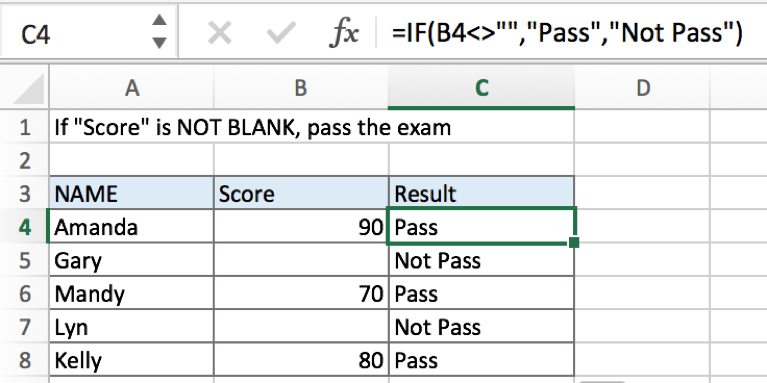In above example, if cell is not blank in B column for a student, we think he passed the exam, formula returns result of true “Pass”, otherwise for a student, if there is no score in B column, we can assume that he didn’t pass the exam, so formula retrieves false value from the two results and returns “Not Pass” for him.

## FORMULA

To test if cell equals a certain text string, the generic formula is:

=IF(A1<>””,”if not blank value”,”blank value”)

Formula in this example:

=IF(B4<>””,”Pass”,”Not Pass”)

## EXPLANATION

In this example, for a student, if cell in “Score” column is not blank, that means he passed the exam, in this case, we want to fill “Pass” in “Result” column. On the other side, if cell is blank, we want to fill “Not pass” for those students who failed the exam. So, there are two results by design, pick which one from the two result is based on the result of the logical test “if cell is blank or not”, in Excel, IF function can handle this case effectively.

IF function allows you to create a logical comparison between your value and reference value (for example “A1>0”), and set true value and false value what you expect to return as test results. IF function returns one of the two results based on logical comparison result.

Syntax: IF(logica_test,[value_if_true],[value_if_false])

To test if B4 is not blank, we can directly create a logical comparison B4<>””. The symbol <> is a logical operator that means “not equal to”. And double quotes “” represents “Empty”, there is no need to add an extra space between “”, but double quotes “” should not be ignored. If missing double quotes, Excel will prompts warning message that the formula you typed contains an error, if you ignore the error, #VALUE! is displayed in cell. Above all, B4<>”” means B4 is not equal to empty.

Besides, for the two arguments “value_if_true” and “value_if_false”, we set “Pass” and “Not Pass” separately. So the formula is: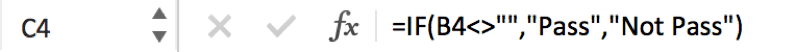In this example, B4 is not a blank cell, logical test B4<>”” is true, so IF function evaluates True value “Pass” to C4.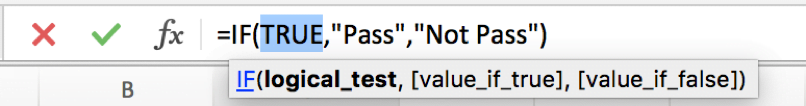For B5, B5 is a blank cell, B5<>”” is false, so IF retrieves False value “Not Pass” to C5.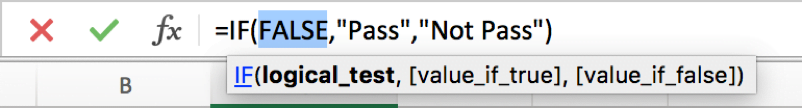In IF, true value and false value can be set as empty string “”, a text, a number or a formula, you can set what you expect to these two values.

## ISBLANK Function

You can also use ISBLANK function to test if a cell is blank or not. In the formula, we can use ISBLANK(B4) to replace original logical test B4<>””. But we should be aware that ISBLANK function is to test if a cell is blank, so it returns true value if it detects that a cell is blank. So, the two results of true and false should be reversed.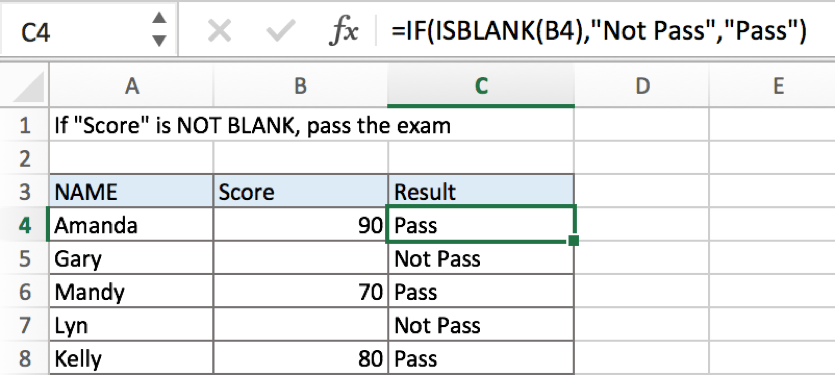If you want to keep the sequence of your two results, you can add NOT function before ISBLANK.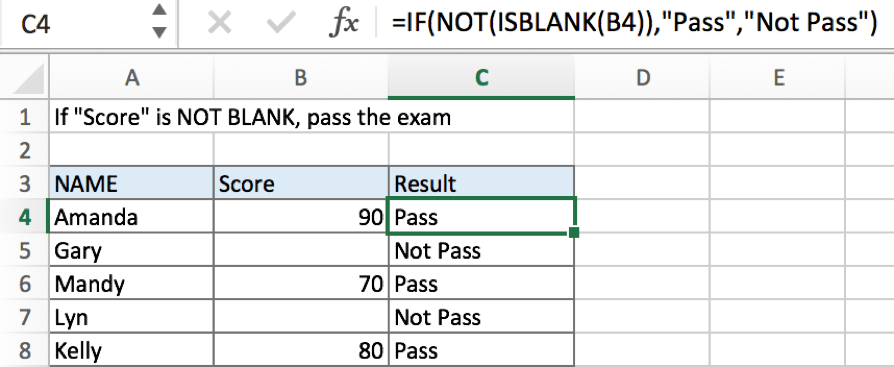### Related Functions

• Excel ISBLANK function
The Excel ISBLANK function returns TRUE if the value is blank or null.The syntax of the ISBLANK function is as below:= ISBLANK (value)…
• Excel IF function
The Excel IF function perform a logical test to return one value if the condition is TRUE and return another value if the condition is FALSE. The IF function is a build-in function in Microsoft Excel and it is categorized as a Logical Function.The syntax of the IF function is as below:= IF (condition, [true_value], [false_value])….

## If Cell is Blank

IF function is frequently used in Excel worksheet to return you expect “true value” or “false value” based on the result of created logical test. If you want to see if a cell is blank or not, and leave some comments for blank cells, you can build a formula with IF function, IF function can return “value if blank” or “value if not blank” based on test “if cell is blank”.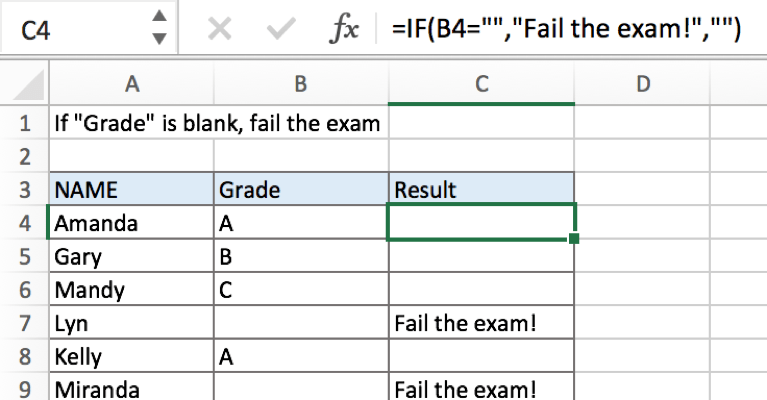## FORMULA

To test if cell equals a certain text string, the generic formula is:

=IF(A1=””,”blank value”,”not blank value”)

Formula in this example:

=IF(B4=””,”Fail the exam!”,””)

## EXPLANATION

In this example, for a student, if cell in “Grade” column is blank, that means he/she failed the exam, and we want to add comment “Fail the exam!” in C column for those students who fail the exam. To return proper value based on “if cell is blank or not”, IF function can handle this case effectively.

IF function allows you to create a logical comparison between your value and reference value (for example “A1>0”), and set true value and false value what you expect to return as test results. IF function returns one of the two results based on logical comparison result.

Syntax: IF(logica_test,[value_if_true],[value_if_false])

To test if B4 is blank, we can directly create a logical comparison B4=“”, “” represents “Empty”, there is no need to add an extra space between “”, but double quotes “” should not be ignored. If missing double quotes, Excel will prompts warning message that the formula you typed contains an error, if you ignore the error, #VALUE! is displayed in cell.

In this example, B4 is not a blank cell, logical test B4=”” returns false to IF functon.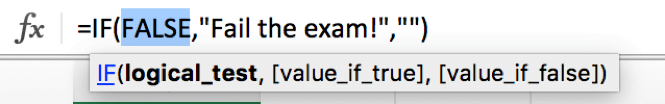As the result is false, IF function retrieves the value from “value if false” argument, in this case it is empty string, so nothing displays in C5. But for B7, B7 is a blank cell, it meets the condition “B7=”””, so IF retrieves true value “Fail the exam!” and return it to C7.

In IF, true value and false value can be set as empty string “”, a text, a number or a formula, you can set what you expect to these two values.

## ISBLANK Function

You can also use ISBLANK function to test if a cell is blank or not. In the formula, we can use ISBLANK(B4) to replace original logical test “B4=”””.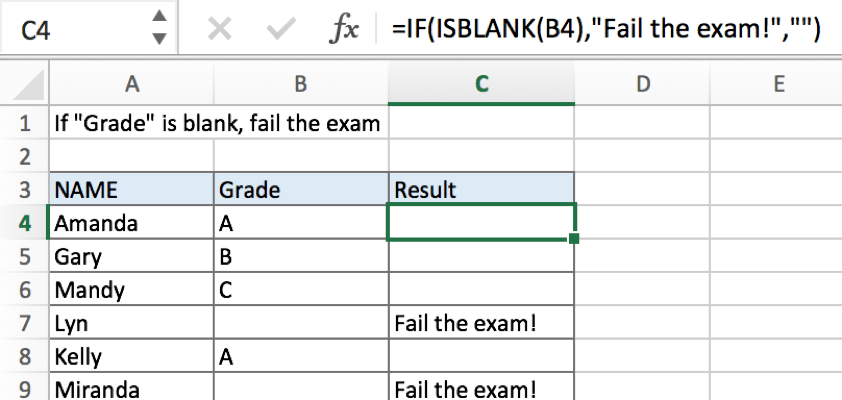### Related Functions

• Excel ISBLANK function
The Excel ISBLANK function returns TRUE if the value is blank or null.The syntax of the ISBLANK function is as below:= ISBLANK (value)…
• Excel IF function
The Excel IF function perform a logical test to return one value if the condition is TRUE and return another value if the condition is FALSE. The IF function is a build-in function in Microsoft Excel and it is categorized as a Logical Function.The syntax of the IF function is as below:= IF (condition, [true_value], [false_value])….

## How to ignore Blank Cells while Concatenating cells in Excel

This post will guide you how to concatenate cells but ignore all blank cells in your worksheet in Excel. How do I concatenate cells but ignore blank cells with a formula in Excel. How to create a concatenate formula to skip blank cells with user defined function in Excel 2013/2016.

Assuming that you have a list of data in range A1:A5 with one or two blank cells, and you want to concatenate those cells with a formula based on Concatenate function but ignore all blank cells. By default, the Concatenate function will combine all selected cells as well as those two blank cells. The below two methods will show you how to concatenate cells but skip all blanks.

## Concatenating Cells but Ignore Blanks with a formula

To concatenate cells but ignore all blank cells in Excel, and you need to use a condition function to check whether the cell has a value or is empty cell. And if the cell is blank, then return nothing; otherwise, return the value of the cell. So you can use the below formula based on the concatenate and the ISBLANK function.

`=CONCATENATE(IF(ISBLANK(A1),"",A1),IF(ISBLANK(A2),"",A2),IF(ISBLANK(A3),"",A3),IF(ISBLANK(A4),"",A4),IF(ISBLANK(A5),"",A5))`

Type the above formula into a blank cell where you want to place the last result, and then press Enter key.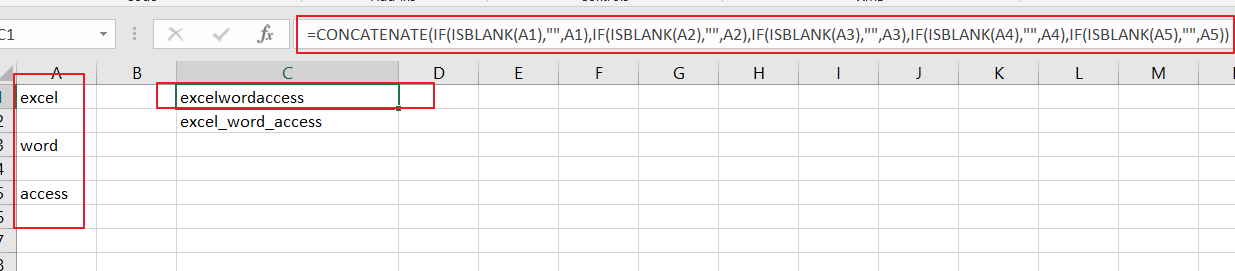## Concatenating Cells but Ignore Blanks with User Defined Function

There is another simple way to concatenate cells but skip all blank cells. And you can create one user defined function with VBA Macro code. Just do the following steps:

#1 open your excel workbook and then click on “Visual Basic” command under DEVELOPER Tab, or just press “ALT+F11” shortcut.#2 then the “Visual Basic Editor” window will appear.

#3 click “Insert” ->”Module” to create a new module.#4 paste the below VBA code into the code window. Then clicking “Save” button.

```Function ConcatenateButBlank(selectArea As Range) As String
For Each oneCell In selectArea: finalResult = IIf(oneCell = "", finalResult & "", finalResult & oneCell & "_"): Next
ConcatenateButBlank = Left(finalResult, Len(finalResult) - 1)
End Function```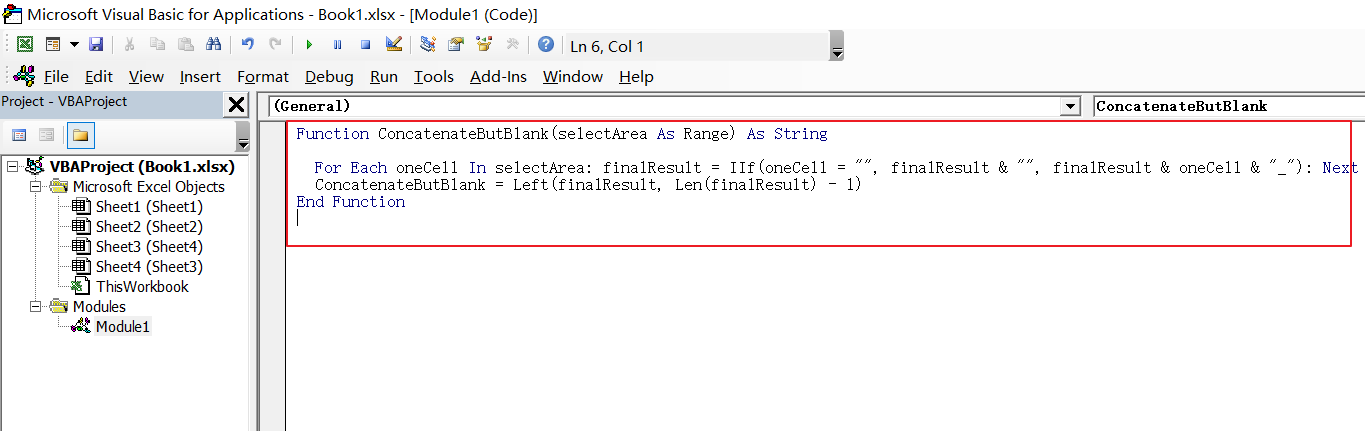#5 back to the current worksheet, then type the following formula in a blank cell, and then press Enter key.

=ConcatenateButBlank(A1:A5)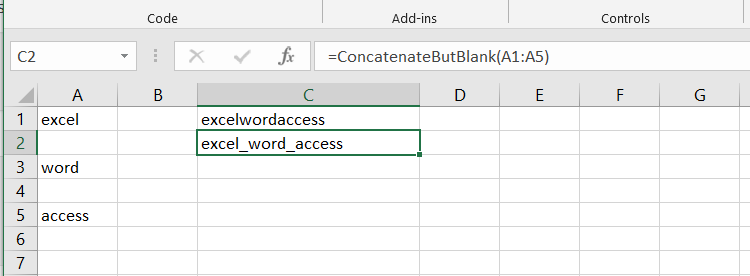### Related Functions

• Excel ISBLANK function
The Excel ISBLANK function returns TRUE if the value is blank or null.The syntax of the ISBLANK function is as below:= ISBLANK (value)…
• Excel IF function
The Excel IF function perform a logical test to return one value if the condition is TRUE and return another value if the condition is FALSE. The IF function is a build-in function in Microsoft Excel and it is categorized as a Logical Function.The syntax of the IF function is as below:= IF (condition, [true_value], [false_value])….

## How to SUM Cells If the Adjacent Cell Match One Criteria in Excel

This post will guide you how to sum a given range of cells that if the adjacent cell meet one criteria in Excel. How do I sum All Cells if its adjacent cell is blank or equals to zero or contain a specific text string value with a formula in Excel.

## SUM Cells If the Adjacent Cell Match One Criteria

Assuming that you have a list of data in range A1:B5, in which contain sales data.  You want to sum all sales values where the adjacent cell equal to product “excel”. How to do it. You can use an Excel Array formula based on the SUM function and the IF function to achieve the result. Like this:

`=SUM(IF(A2:A6="excel",B2:B6,0))`

You need to type this formula into a blank cell and press Ctrl + Alt + Enter keys on your keyboard to change the normal formula as array formula.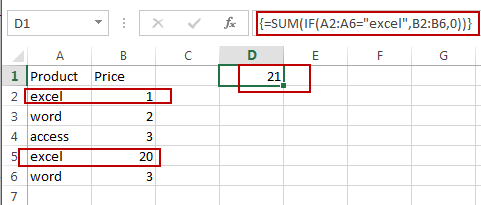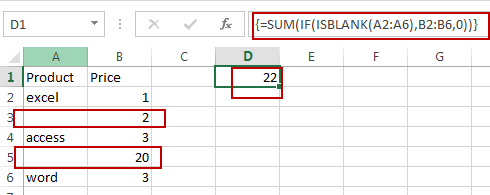Note: the A2:A6 range is the data range which contain the criteria that need to match. And the B2:B6 is a range which have sales values that you want to sum based on the matched criteria.

If you want to sum all values if the adjacent cell is a blank cell in your data, you can use another array formula based on the SUM function, the IF function and the ISBLANK function to achieve the result. Like this:

`=SUM(IF(ISBLANK(A2:A6),B2:B6,0))`

Type this formula into a blank cell and press Ctrl + Alt + Enter keys on your keyboard.

### Related Functions

• Excel SUM function
The Excel SUM function will adds all numbers in a range of cells and returns the sum of these values. You can add individual values, cell references or ranges in excel.The syntax of the SUM function is as below:= SUM(number1,[number2],…)…
• Excel IF function
The Excel IF function perform a logical test to return one value if the condition is TRUE and return another value if the condition is FALSE. The IF function is a build-in function in Microsoft Excel and it is categorized as a Logical Function.The syntax of the IF function is as below:= IF (condition, [true_value], [false_value])….
• Excel ISBLANK function
The Excel ISBLANK function returns TRUE if the value is blank or null.The syntax of the ISBLANK function is as below:= ISBLANK (value)…

## How to Find the First or Last Non-blank Cell in a Row or Column in Excel

This post will guide you how to find the first non-blank cell in a row or column or a range in Excel. How do I get the value of the last non-blank cell in a row or column using formula in Excel.

## Get First Non-blank Cell Value

Assuming that you have a list of data in range A1:A5, and you want to find the first non-blank cell in a given range of cells in Excel. How to do it. You can use a formula based on the INDEX function and the MATCH function to get the first non-blank value in a one-column range in Excel. Here is the formula you can use:

`=INDEX(A1:A5,MATCH(TRUE,INDEX((A1:A5<>0),0),0))`

Type this formula into a blank cell and press Enter key on your keyboard. You would get the first non-blank cell value from your given range A1:A5.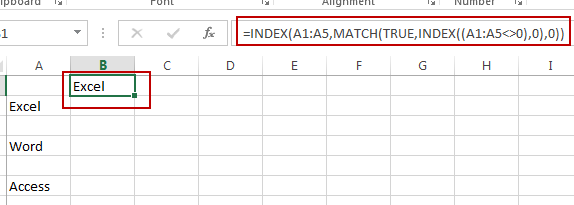Or you can use an array formula based on the INDEX function, the MATCH function and the ISBLANK function to achieve the same result of extracting the first non-blank cell value from a given range in Excel. Like this:

`=INDEX(A1:A5,MATCH(FALSE,ISBLANK(A1:A5),0))`

Type this formula into a blank cell and press Ctrl + Shift + Enter keys on your keyboard to make your formula as array formula.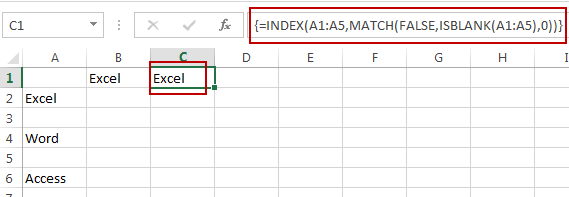## Get Last Non-blank Cell Value

If you want to find the value of the last non-blank cell in a row or column in Excel, you can use a formula based on the LOOKUP function to achieve it. Here is the formula we are using is:

`=LOOKUP(2,1/(A1:A5<>""),A1:A5)`

Type this formula into a blank cell and press Enter key on your keyboard. You would get the last non-blank cell value from your given range A1:A5.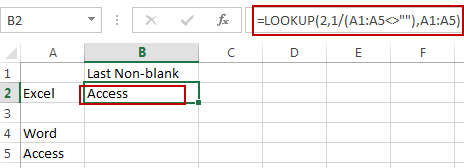### Related Functions

• Excel LOOKUP function
The Excel LOOKUP function will search a value in a vector or array.The syntax of the LOOKUP function is as below:= LOOKUP (lookup_value, lookup_vector, [result_vector])…
• Excel INDEX function
The Excel INDEX function returns a value from a table based on the index (row number and column number)The INDEX function is a build-in function in Microsoft Excel and it is categorized as a Lookup and Reference Function.The syntax of the INDEX function is as below:= INDEX (array, row_num,[column_num])…
• Excel MATCH  function
The Excel MATCH function search a value in an array and returns the position of that item.The MATCH function is a build-in function in Microsoft Excel and it is categorized as a Lookup and Reference Function.The syntax of the MATCH function is as below:= MATCH  (lookup_value, lookup_array, [match_type])….
• Excel ISBLANK function
The Excel ISBLANK function returns TRUE if the value is blank or null.The syntax of the ISBLANK function is as below:= ISBLANK (value)…

## Ignoring Blank or Zero Cells with Conditional formatting

This post will guide you how to make conditional formatting ignore blank cells or zero cell in Excel. How do I force blank cells or zero cells to be ignored in conditional formatting in Excel. How to ignore blank cells or blank cells when applying conditional formatting in Excel.

## Ignoring Blank with Conditional Formatting

When you create conditional formatting rules for a list of selected data, and you want to ignore all Blank cells, How to achieve it. Just do the following steps:

#1 select the source data that you want to apply the conditional Formatting.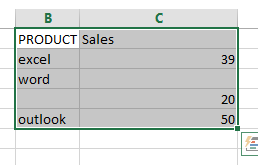#2 go to HOME tab, click Conditional Formatting command under Styles group. And select New Rule from the popup menu list. And the New Formatting Rule dialog will open.

#3 select Use a formula to determine which cells to format in the Select a Rule Type section.

#4 type the following formula into the Format values where this formula is true text box. Click Ok button.

=ISBLANK(B1)=TRUE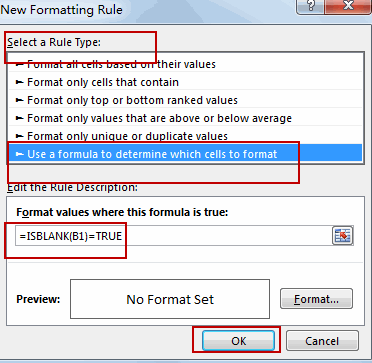Note: the B1 is the first cell of the selected range of cells.

## Ignoring zero with Conditional Formatting

If you want to ignore zero values with conditional Formatting, you can do the following steps:

#1 select the range of cells C2:C5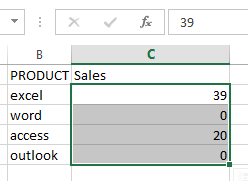#2 go to HOME tab, click Conditional Formatting command under Styles group, and select New Rule, and the New Formatting Rule dialog will open.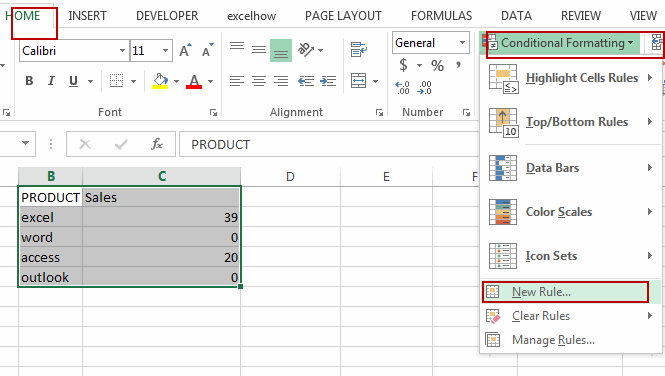#3 select Use a formula to determine which cells to format in the Select a Rule Type section.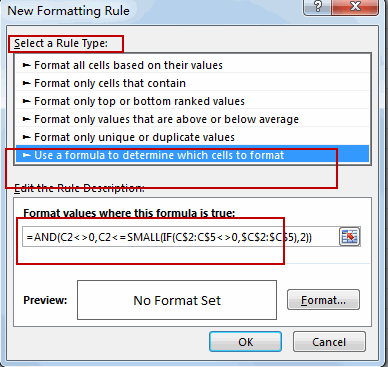#4 type the following formula into the Format values where this formula is true text box.

=AND(C2<>0,C2<=SMALL(IF(C\$2:C\$5<>0,\$C\$2:\$C\$5),2))

#5 click Format button, and switch to Fill tab in Format Cells dialog, select one color as the background color. Click Ok button.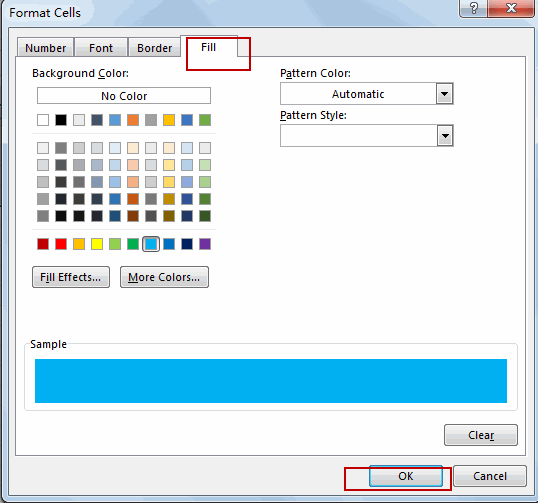#6 click Ok button. You will see that all zero values are ignored by conditional formatting.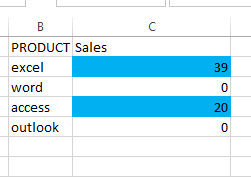### Related Functions

• Excel ISBLANK function
The Excel ISBLANK function returns TRUE if the value is blank or null.The syntax of the ISBLANK function is as below:= ISBLANK (value)…
• Excel IF function
The Excel IF function perform a logical test to return one value if the condition is TRUE and return another value if the condition is FALSE. The IF function is a build-in function in Microsoft Excel and it is categorized as a Logical Function.The syntax of the IF function is as below:= IF (condition, [true_value], [false_value])….
• Excel SMALL function
• The Excel SMALL function returns the smallest numeric value from the numbers that you provided. Or returns the smallest value in the array.The syntax of the SMALL function is as below:=SMALL(array,nth) …
• Excel AND function
• The Excel AND function returns TRUE if all of arguments are TRUE, and it returns FALSE if any of arguments are FALSE.The syntax of the AND function is as below:= AND (condition1,[condition2],…)…

## Excel IF formula with NOT logical function

This post will guide you how to use excel if formula with NOT logical function in Microsoft excel.

## NOT Logical Function

The syntax of NOT logical function is as follow:

`=NOT(logical_value)`

The NOT logical function will return the reversed logical value. If the logical_value is TRUE, then FALSE is returned. If the logical_value is FALSE, then TRUE is returned. Let’s see the below two examples:

```=NOT(FALSE)
=NOT(2+1=3)```

Both of the above excel formulas will return TRUE.

## Excel IF Formula Combining With NOT

If you want to check several test conditions in an excel formula,then take different actions. You can use NOT function in combination with the AND or OR logical function in excel IF function.

Let’s see the following excel if formula:

`=IF(NOT(OR(B1="red",B1="black")),TRUE,FALSE)`

In the above formula, the excel formula will exclude both red and blank colors. It will check cells in column B if the values is NOT red or blank. TRUE or FALSE will be returned.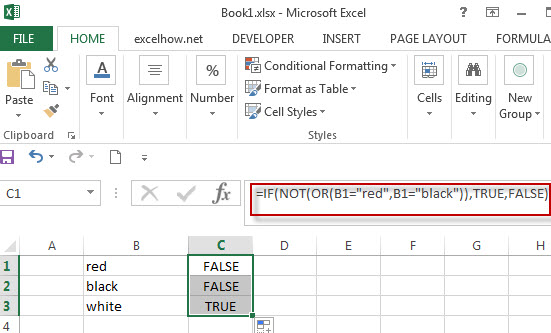Actually, we also can use the Not equal to (<>) logical operator to achieve the same requirement , For example, we can write down the following excel IF formula to reflect the above logic test.

`=IF(AND(B1<>”red”,B1<>”black”)),TRUE,FALSE)`

## NOT Function combining with ISBLANK function

As you know, the function ISBLANK(B1) will return TRUE if the cell B1 is blank. If we use NOT function to enclose ISBLANK(B1) function, it will reverse the result, if the cell is blank, then returns FALSE.

Let’s create a nested IF statement with the NOT and ISBLANK functions as follow:

`=IF(NOT(ISBLANK(B1)),B1*5,””)`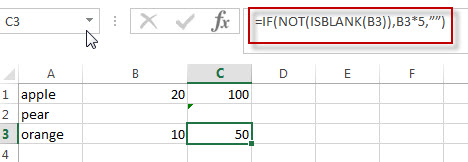For above IF statement, IF the cell B1 is not blank, then multiply the number in B1 by 5.we can enter the above formula into cell C1, and then drag the Fill Handle to the range C1:C3.it will apply for the other cells for this formula.

### Related Functions

• Excel OR function
The Excel OR function returns TRUE if any of the conditions are TRUE in logic test. Otherwise, it returns FALSE.
• Excel AND function
The Excel AND function returns TRUE if all of arguments are TRUE, and it returns FALSE if any of arguments are FALSE.The syntax of the AND function is as below:= AND (condition1,[condition2],…) …
• Excel IF function
The Excel IF function perform a logical test to return one value if the condition is TRUE and return another value if the condition is FALSE….
• Excel nested if function
The nested IF function is formed by multiple if statements within one Excel if function. This excel nested if statement makes it possible for a single formula to take multiple actions…
• Excel ISBLANK function
The Excel ISBLANK function returns TRUE if the value is blank or null.The syntax of the ISBLANK function is as below:= ISBLANK (value)…
• Excel NOT function
The Excel NOT function returns the opposite of a given logical or Boolean value. For example, if you supplied with the value TRUE, the NOT function will return FALSE; If you supplied with the value FALSE, and the NOT function will TRUE. The syntax of the NOT function is as below:=NOT(logical)…

## Excel Nexted IF Functions (Statements) Tutorial (15 IF Formulas examples)

This tutorial will guide you how to use nested Excel IF function  (include multiple If statements in excel formula) with syntax and provide about 15 nested IF formula examples with the detailed explanation in Microsoft excel.

## Description

The Excel IF function perform a logical test to return one value if the condition statement is TRUE and return another value if the condition statement is FALSE. The IF function is a build-in function in Microsoft Excel and it is categorized as a Logical Function.

The excel if function just only test one condition and if you want to deal with more than one condition and return different actions depending on the result of the tests,  then you need to include several IF statements (functions) in one excel IF formula, these multiple IF statements are also called Excel Nested IF formula(Nested IFs). It’s also similar with IF-THEN-ELSE statement.

The nested IF function is formed by multiple if statements within one Excel if function. This excel nested if statement makes it possible for a single formula to take multiple actions.

The most recent versions of Excel (Excel 2016, Excel 2013, Excel 2010 and Excel 2007) allow 64 IF functions (statements) in one formula, and it was only possible up to 7 nested IF functions (statements) in Excel 2003 and lower version.

## Syntax

The syntax of Nested IF function is as below:

`=IF(Condition_1,Value_if_True_1,IF(Condition_2,Value_if_True_2,Value_if_False_2))`

Where the Nested IF function argument is:

Condition_1 –  The condition that you want to test  in the first IF statement.

Value_if_True_1 – The value that is returned if first IF statement is True. If the condition_1 return False, then move into the next IF function.

Condition_2 – The condition that you want to test in the second IF statement.

Value_if_True_2 – The value that is returned if second IF statement is True.

Value_if_False_2 – The value is returned if second IF statement is False.

This is equivalent to the following IF THEN ELSE statement:

```IF Condition_1 THEN
Value_if_True_1
ELSEIF Condition_2
Value_if_True_2
ELSE
Value_if_False_2
END IF```

## Examples of Nested IF function (Statement) in Excel

The below examples will show you how to use Excel Nested IF function with the detailed explanation of their syntax and logic.

Example 1#  The most basic Nested IF function with one level of nesting

If you want to write a nested if function to test the following calculation logic for assigning value in the cell A1.

```IF A1 =="excelhow" THEN
return "excel"
ELSEIF A1 == "excelhow.net" THEN
return "MS excel"
ELSE
return "MS"
END IF```

we can write a nested IF function based on the above logic as follows:

`=IF(A1="excelhow", "excel", IF(A1="excelhow.net","MS excel","MS"))`

In above Nested IF formula, the nested if function is shown with bold style and it is inside the outer IF function. we can see that if A1 is not equal to the “excelhow“, then the second nested  IF function will be test. and if second IF condition statement return FALSE, then the entire IF function will return “MS” value.

Example 2#  The Nested IF function with two levels of nesting

Assuming that you want to test more than one condition statement in the above nested if function, add one condition to test if the value of the cell A1 reference is equal to “www.excelhow.net” , If TRUE, then return “Microsot excel“.

The calculation Logic is as below:

```IF A1 =="excelhow" THEN
return "excel"
ELSEIF A1 == "excelhow.com" THEN
return "MS excel"
ELSEIF A1 == "www.excelhow.net" THEN
return "Microsoft excel"
ELSE
return "MS"
END IF```

we can add one more IF statement inside the second IF function in the above excel nested if formula in example1. let’s see the below nested if function with tow level nesting:

`=IF(A1="excelhow", "excel", IF(A1="excelhow.com","MS excel",IF(A1="www.excelhow.net","Microsoft excel","MS")))`

In the above nested excel IF formula, the first nested if function is marked with red color, and the second nested excel if function is marked with blue color.

If the both first and second conditions are False and the third IF condition will be check, IF A1 is equal to “www.excelhow.net” , then return “Microsoft excel“, or the entire nested IF formula will return “MS“.

Example 3#  Describes the each IF function contained in the nested IF function

​We will use one typical example of excel nested if function to describe each IF function included in the nested if function.

Assuming that you need to assign a grade based on a score with the following test conditions:

Let’s write a nested if function based on the above logic as follows:

`=IF(A1>=80, "excellent", IF(A1>=60, "good", IF(A1>0, "bad", "no valid score")))`

For the above excel if formula, lets describe it for each IF function statement.

1# IF Cell A1 is greater than or equal to 80, then the formula will return “excellent” or move to the second If function.

2# If Cell A1 is greater than or equal to 60, then the formula will return  “good” or move to the third IF function

3# IF Cell A1 is greater than 0, then the formula will return “bad”, or the IF function will return “no valid score”.

Example 4#  Describes each If function in the excel Nested IF Statement (another simple example of if function)

Let’s describe the below Nested IF Function example:

`=IF(A1<=6,60, IF(A1<=8,80,IF(A1<=10,100,200)))`

a) If cell A1 is equal to 6 or less than 6, then return value 60 in cell C1. Let’s see below screenshot.

b) If Cell A1 is greater than 6 and less or equal to 8, then retrun value 80 in Cell C1.

c) If cell A1 is greater than 8 and less than or equal to 10, then return value 100 in cell C1.

d) If cell A1 is greater than 10 , then the Nested if function will return the last value “200”in cell C1.

Example 5#  Excel Nested IF function with arithmetic operator (+, -, * , /)

Assuming that you want to write a Nested If function to reflect the following logic tasks:

a) IF Cell A1 is less than 10, then multiply by 10.

b) IF Cell A1 is greater than or equal to 10 but less than 20, then add 20

c) IF Cell A1 is greater than or equal to 20 but less than 30, then minus 20

d) IF Cell A1 is greater than or equal to 30 but less than 50, then divided by 20

The nested IF formula is as follows:

`=IF(A1<10,A1*10,IF(A1<20,A1+20,IF(A1<30,A1-20,IF(A1<50,A1/20))))`

a1) if Cell A1 is less 10 (A1=5), then the first If condition matched and will take multiply action, A1 * 10=5*10=50, so it will return 50 in the cell C1

b1) if Cell 10<=A1<20 (A1=15), then the second if condition matched and will take add action, A1+20=15+20=35, so it will return 35 in the cell C1.

c1) if Cell 20<=A1<30(A1=25), then the third if condition matched and will take minus action, A1-20=25-20=5, so it will return 5 in the cell C1.

d1) if Cell 30<=A1<50 (A1=35), then the forth if condition matched and will take divide action, A1/20=35/20=1.75, so it will return 1.75 in the cell C1.

Example 6#  Excel Nested IF function with logical function –AND

Assuming that you need a nested if function to reflect the following logic:

a) IF A1+B1 is less than 10, then return 10

b) IF A1+B1 is greater than 10 but less than or equal to 20, then return 20

c) IF A1+B1 is greater than 20 but less than or equal to 30, then return 30.

d) IF A1+B1 is greater than 30, then return 200.

Let’s write the following nested IF formula in the cell C1:

`=IF(A1+B1<10,10, IF(A1+B1<=20,20, IF(A1+B1<=30,30,200)))`

The above formula just use basic nested IF function syntax, we also can use logic function to re-write it, the nested if formula with AND function is as follows:

`=IF((A1+B1)<10,10,IF(AND((A1+B1)>10,(A1+B1)<=20),20, IF(AND((A1+B1)>20,(A1+B1)<=30),30,200)))`

The above nested IF formula combined with two AND function.

In the second IF Statement, AND((A1+B1)>10,(A1+B1)<=20) will check if 10<A1+B1<=20, If TRUE, then the formula will return 20.

In the third IF Statement, AND((A1+B1)>20,(A1+B1)<=30) will check if 20<A1+B1<=30, If TRUE, then the formula will return 30.

Example 7# Excel Nested IF function with logical function –OR

Assuming that you need a nested if function to reflect the following logic:

a) IF Cell A1=5, return A1/B1

b) IF Cell A1=10, return A2/B2

c) IF Cell A1=15 or A1=20, return A3/B3

In Cell C1, we can write the below nested if formula based on the above conditions.

`=IF(A1=5,A1/B1,IF(A1=10,A2/B2,IF(OR(A1=15,A1=20),A3/B3)))`

One OR function be used in the above excel nested if function, it will check if A1=15 or A1=20, if TRUE, then return A3/B3.

Example 8#  Excel nested if function with text and logical function AND

Wrote a nested if function with text to reflect the following logic:

a) If Cell A1=”E” and Cell B1=5, then return “Excel”

b) If Cell A1=”P” and Cell B1=3, then return “PPT”

c) If Cell A1=”W” and Cell B1=4, then return “Word”

d) Else return “Access”

In Cell C1, try to enter into the following excel nested If formula with AND function:

`=IF(AND(A1="E",B1=5),"Excel", IF(AND(A1="P",B1=3),"PPT", IF(AND(A1="W",B1=4),"Word","Access")))`

Example 9#  Excel nested if function with ISBLANK function and logical function AND

a) If you want to wrote a nested if function with ISBLANK function and logical function AND to reflect the following logic:

b) If both Cell A1 and Cell B1 are empty, then return “”

c) If only Cell A1 is empty, then return B1-today()

d) If both two cells A1 and B1 are not empty, then return “excel” string.

In Cell C1, use the following excel nested If formula with ISBLANK and AND function:

`=IF(AND(ISBLANK(A1),ISBLANK(B1)),"",IF(ISBLANK(A1),B1-TODAY(),"excel"))`

Example 10#  Using nested IF functions to check grade level based on student’s score(multiple IF statements)

The logic is as below:

We will write a nested If function that reflect the above logic, and will check if the score is below 50, If TRUE, it is considered as “Fail”. If FALSE, move into the next IF statement to test if the score is between 51 and 60 and it is considered as “Grade C”. If False, we will move into another IF statement to check if the score is between 61 and 80, IF True and it is considered as “Grade B”. If FASLSE, just check the rest conditions.

We can use a nested if formula as follows:

`=IF(B2<=50,"Fail",IF(AND(B2>=51, B2<=60), "Grade C", IF(AND(B2>=61, B2<=80),"Grade B",IF(AND(B2>=81,B2<=95),"Grade A", "Grade A+"))))`

Example 11#  Nested IF function for checking two Empty Cells

Let’s see the below image a product table of a company (need to create an excel table firstly):

a) If we need to check both “Price” cell and “Quantity” cell are empty, If True, then return empty. If the only “price” cell is empty only, IF True, return empty.

b) If the only “Quantity” cell is empty, IF True, return empty.

c) If both “price” and “Quantity” are not empty, then return multiply Price * Quantity as subtotal value.

So To check both “Price” and Quantity cells, we can use table header name as condition variable to test each Price cells or Quantity cells, so we can write the nested if formula as follows:

`=IF([Price]="","",IF([Quantity]="","",[Price]*[Quantity]))`

Just using the above excel if formula in the subtotal cells, the formula will check the first IF statement if Price Cell is empty, IF TRUE, then will return empty (“”) in the subtotal cell. IF FALSE, then move to the next IF statement and so on. Last, IF neither cell is empty, then will return the value of multiply [Price]*[Quantity] in subtotal cell.

Of course, we can use another nested if function to achieve the above calculation logic (easy to understand).

`=IF(ISBLANK(B2),"",IF(ISBLANK(C2),"", B2*C2))`

OR

`=IF(B2="","",IF(C2="","", B2*C2))`

## Nested IF Functions Order

There is one important thing that need us keep in mind when write Excel Nested IF Function, it is the order of nested IF function. It can nested up to 64 If statements, and how to order multiple IF condition statements, it is key point. Or the wrong result will be returned. The point is that excel nested if function will test the first if condition in the order, once any condition is met, and the subsequent if conditions will not be checked.

So let’s remember the below rules while writing excel nested if function:

• The most important condition First or Harder Test First

Let’s see the below example what it means:

Example 12#

There are two test conditions in the following excel nested if function:

`=IF(B1>=90,"excellent",IF(B1>=60,"good","bad"))`

When using this formula in the cell B3, If the amount in cell B1 is 95, then “excellent” would be returned. because it is greater than 90. And the second IF condition will not be evaluated.

However, if the order of nested if statment are reversed as follows:

`=IF(B1>=60,"good",IF(B1>=90,"excellent","bad"))`

The above formula would check for the condition B1>=60 first, if the amount in cell B1 is 95,  then the value “good” would be returned in cell B3. Because the Cell B1 match the first test condition, and it will not check the second if condition and will return the incorrect result.

## Nested IF Function Alternatives

To make your excel formulas more efficiency and fast, you can try to use the following alternatives to excel nested if function.

1)
Excel nested if function can be easily replaced with the VLOOUP, Lookup, INDEX/MATCH or CHOOSE functions.

Example 13#  Use VLookup function instead of nested IF function

Nested IF function:

`=IF(D1=39,"excelhow.net", IF(D1=45,"two",IF(D1=34,"google.com","")))`

Vlookup function:

`=VLOOKUP(39,A1:B3,2,FALSE)`

Example 14#  Use CHOOSE function instead of nested if function

Nested If unction:

`=IF(B1>=60,"good","bad")`

Using CHOOSE function as follows:

`=CHOOSE(IF(B1<60,1,2),"bad","good")`

2) Use IFS instead of nested if function

3) Use the CONCATENATE function or the concatenate operator (&).

Example 15#

Nested IF function:

`=IF(B1="A","excellent",IF(B1="B","good","bad"))`

Using CONCATENATE function as follows:

`=CONCATENATE(IF(B1=”A”,”excellent”,””),IF(B1=”B”,”good”,””),IF(B1=”C”,”bad”))`

Question1: Is there any tool to help write Excel formulas and nested Ifs?

This is an Excel formula with nested IF statements:

`=IF((B2="East"),4,IF((B2="West"),3,IF((B2="North"),2,IF((B2="South"),1,""))))`

To essentially accomplish this:

```If cell B2 = "East"
return "4"
ElseIf cell B2 = "West"
return "3"
ElseIf cell B2 = "North"
return "2"
ElseIf cell B2 = "South"
return "1"
Else
return ""```

Can Excel formulas be written in such a “more readable” manner and converted to the official syntax? Is there any tool to help write Excel formulas?

Answer: Excel Formula Formatter add-in by Rob van Gelder, mentioned at Daily Dose of Excel.

Excel’s formula bar ignores line feeds and white space, so you can Alt+Enter and spacebar to format the formulas however you like. I’ve tried it and I quickly stopped doing it. Too much spacebar-ing, especially if you need to edit.

Question2: I am working on an excel file, and i am trying to use a nested if formula to achieve what i would like.

i have two columns:A B.  And the condition is this: if the value in a2=a3, then check if the minus of b2 and b3 is certain value, and if it is, put a yes, else put a no. this will iterate till the end of the excel file.

so far here is what i have. not sure how to use the excel formulas. any help is much appreciated.

`if(a2=a3,b2-b3=5 or b2-b3=-5 or b2-b3=20 or b2-b3=-20, "yes", "no")`

Answer: you should be able to use the OR function within your nested if formula to test for “B2-B3=5,B2-B3=-5,B2-B3=20,B2-B3=-20” as follows:

`=IF(A2=A3,IF(OR(B2-B3=5,B2-B3=-5,B2-B3=20,B2-B3=-20),"yes","no"),"no")`

### Related Functions

• Excel IF function
The Excel IF function perform a logical test to return one value if the condition is TRUE and return another value if the condition is FALSE….
• Excel And function
The Excel AND function returns TRUE if all of arguments are TRUE, and it returns FALSE if any of arguments are FALSE…
• Excel Concat Function
The excel CONCAT function combines 2 or more strings or ranges together. The syntax of the CONCAT function is as below: =CONCAT (text1,[text2],…) …
• Excel Vlookup Function
The Excel VLOOKUP function lookup a value in the first column of the table and return the value in the same row based on index_num position.The syntax of the VLOOKUP function is as below:= VLOOKUP (lookup_value, table_array, column_index_num,[range_lookup])…
• Excel Choose Function
The Excel CHOOSE function returns a value from a list of values. The CHOOSE function is a build-in function in Microsoft Excel and it is categorized as a Lookup and Reference Function.The syntax of the CHOOSE function is as below:=CHOOSE (index_num, value1,[value2],…)…
• Excel ISBLANK function
The Excel ISBLANK function returns TRUE if the value is blank or null.The syntax of the ISBLANK function is as below:= ISBLANK (value)…

## Excel ISBlank Function

This post will guide you how to use Excel ISBLANK function with syntax and examples in Microsoft excel.

## Description

The Excel ISBLANK function returns TRUE if the value is blank or null.

The ISBLANK function is a build-in function in Microsoft Excel and it is categorized as an Information Function.

The ISBLANK function is available in Excel 2016, Excel 2013, Excel 2010, Excel 2007, Excel 2003, Excel XP, Excel 2000, Excel 2011 for Mac.

## Syntax

The syntax of the ISBLANK function is as below:

= ISBLANK (value)

Where the ISBLANK function argument is:
Value -This is a required argument. The value that you want to test.

## Example

The below examples will show you how to use Excel ISBLANK Function to test if the value is blank, if so, return TRUE.

#1 = ISBLANK (B1)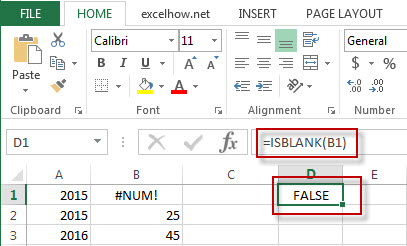### Related Functions

• Excel ISNUMBER Function
The Excel ISNUMBER function returns TRUE if the value in a cell is a numeric value, otherwise it will return FALSE.The syntax of the ISNUMBER function is as below:=ISNUMBER (value)
• Excel ISERR Function
The Excel ISERR function returns TRUE if the value is any error value except #N/A.The syntax of the ISERR function is as below:= ISERR (value)…
• Excel ISError Function
The Excel ISERROR function used to check for any error type that excel generates and it returns TRUE for any error type, such as: #N/A, #VALUE!,#REF!,#DIV0!, #NAME?, etc. The syntax of the ISERROR function is as below:= ISERROR (value)…
• Excel ISNA Function
The Excel ISNA function used to check if a cell contains the #N/A error, if so, returns TRUE; otherwise, the ISNA function returns FALSE. The syntax of the ISNA function is as below:=ISNA(value)…
• Excel ISTEXT Function
The Excel ISTEXT function used to check if a value is text. If so, returns TRUE; if the text is not text, the function will return FALSE. The syntax of the ISTEXT function is as below:=ISTEXT(value)…
• Excel ISNONTEXT Function
The Excel ISNONTEXT function used to check if a value is text. If so, returns FALSE; if the text is not text, the function will return TRUE. The syntax of the ISNONTEXT function is as below:=ISNONTEXT (value)…
• Excel ISREF Function
The Excel ISREF function used to check if a value is a valid reference. If so, returns TRUE; if the value is not a reference, the function will return FALSE. The syntax of the ISREF function is as below:=ISREF(value)…